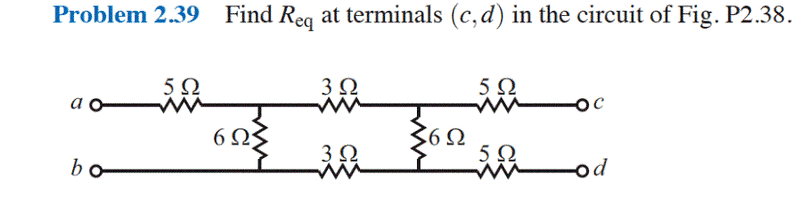# Resistor problem

For this problem I am just wondering if it is proper to "imagine" there is a voltage source from a to b so that one may say the 5 ohm resistor right after a is in parallel with the 6 ohm resistor connecting the two wires. If not, how would I go about solving this?Thank you in advance.

## Answers and Replies

mfb
Mentor
Why a and b? You are looking for the equivalent resistance between c and d. There you can add a voltage source.

There is no 5 ohm resistor in parallel to a 6 ohm resistor indepenent of the points you consider.

Hesch
Gold Member
one may say the 5 ohm resistor right after a is in parallel with the 6 ohm resistor connecting the two wires.
You could substitute the two resistors with a Thevenin-equivalent with a voltage source, VT and a resistor, RT:

VT = Vab * 6Ω / ( 5Ω + 6Ω )

RT = 5Ω || 6Ω

. . . and so on.
If not, how would I go about solving this?
Use Kirchhoffs laws ( KVL and KCL ), set Vab = 100V, then calculate Vcd when cd is unloaded.
Then calculate ( KVL and KCL ) Icd when cd is short circuited.
Req = Vcd / Icd

mfb
Mentor
Are a and b connected to anything? The problem statement does not mention this.
If they are not connected, the problem can be solved with parallel and series resistances only.
If they are connected, the posted problem statement is incomplete.

CWatters
Science Advisor
Homework Helper
Gold Member
bigFlorida..... You might be over thinking the problem.

a and b are open circuit so the 5R can be ignored.

The approach you suggested could be applicable _if_ there was a voltage source connected but you can't just add one.